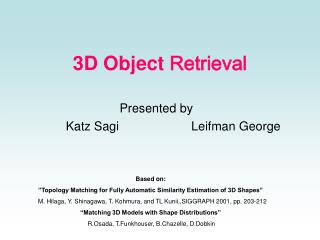# 3D Object Retrieval - PowerPoint PPT PresentationDownload Presentation3D Object Retrieval

3D Object Retrieval
Download Presentation## 3D Object Retrieval

- - - - - - - - - - - - - - - - - - - - - - - - - - - E N D - - - - - - - - - - - - - - - - - - - - - - - - - - -
##### Presentation Transcript

1. 3D Object Retrieval Presented by Katz Sagi Leifman George Based on: ”Topology Matching for Fully Automatic Similarity Estimation of 3D Shapes” M. Hilaga, Y. Shinagawa, T. Kohmura, and TL Kunii,,SIGGRAPH 2001, pp. 203-212 “Matching 3D Models with Shape Distributions” R.Osada, T.Funkhouser, B.Chazelle, D.Dobkin

2. 3D Objects Retrieval – Why? • Improved modeling tools • Improved scanning devices • Fast and cheap CPUs, Gfx HW • Large databases • E-commerce • Medicine • Entertainment • Molecular biology • Manufacturing

3. 2D Object Boundaries Features Occlusion Camera dependent Noise Simple Contour representation 3D Problematic Surface Representation Ambiguous Triangulation No Features Occlusion, shadows, noise 3D vs. 2D Retrieval

4. Common Approach • Preprocess Stage: • Objects Normalization (optional) • Signature for each object • Compact • Capture the object properties • Comparable • Signature Comparison: • Coarse-to-Fine (optional) • Fast DB Object signatures Query Queryprocess Signatures Comparison Similarobjects Object

5. Signature Properties • What do we want from good signature? • Robustness to resampling and simplification • Translation, Orientation, Scale Invariance • Possible Solutions • Preprocess: Object Normalization • Automatically embedded in the key by definition = =

6. Object Normalization using Moments Translation: (m100, m010, m001) – center of mass Rotation, Scale: ∆(1,1) - main axis scale U – Rotation Matrix

7. Different Methods • Octrees • Probability Shape Distributions • Distances to Enclosing Sphere • Reeb Graphs

8. Octrees Signature: • Each object is represented by Octree • White, black, gray (gray level) Signature Comparison: • Coarse-to-Fine search (tree depth)

9. Probability Shape Distributions Several types of signatures: • A3: angle between three random surface points • D1: distance from fixed point to random point • D2: distance between two random surface points X-axis: D2 distance Y-axis: Probability of that distance Signature Comparison: L1,L2,L∞for PDF and CDF

10. Distances to Enclosing Sphere • Signature: • Sphere is evenly sampled • For each sphere sample min. distance to object calculated Signature Comparison: L1,L2,L∞

11. Topology MatchingUsing MRGs

12. The Idea • The signature: • Multiresolutional Reeb Graphs (MRGs) • Represents the skeletal and topological structure of a 3D shape at various levels of resolution • Constructed using a continuous function on the 3D shape. • Correspondence between the parts of objects. • Invariant to transformations and non-rigid deformations • The search: • coarse to fine

13. How to “Reeb” an Object • We’ll create a simple reeb graph using height function • μ - height of the point V: μ(V(x,y,z))=z

14. MultiResolutional Reeb Graph(MRG) • A series of Reeb graphs at various levels of detail

15. The Construction of the MRG • Define the following notation: • R-node: A node in an MRG. • R-edge: An edge connecting R-nodes in an MRG. • T-set: A connected component of triangles in a region • µn -range: A range of the function µn concerning an R-node or a T-set.

16. The Construction of the MRG cont. • The domain of µn is divided onto K µn-ranges: • R0=[0,1/K),R1=[1/k,2/k)….Rk-1=[(K-1)/K,1) • Note: The example uses the height function for the convenience of explanation

17. The Construction of the MRG cont. • Subdivision • Interpolate the position of two relevant vertices in the same proportion as their value of µn(v)

18. The Construction of the MRG cont. • Calculate T-sets • Connect R-nodes

19. The Construction of the MRG cont. • Construct MRG • fine-to-coarse (reverse)

20. Defining µ for Topology Matching • Height function is not appropriate • not invariant to transformations. • Use a geodesic distance • Not invariant to scale: • Normalize [0,1]:

21. Examples of the Distribution of the Function µ • More asymmetric shapes have a wider range for µn(v) • Sphere • constant value of µn(v)=0

22. Matching • Assign 2 attributes for each node (m) in the finest resolution • Area • Length

23. Matching cont. • Define ‘+’: • At coarse resolution • Similarity (0<=w<=1) • To satisfy: • Define

24. Matching cont.

25. “Topology Matching” Added Value • Topology matching can be used to find correspondence between meshes • Problem: The algorithm does Not distinguish between Left and right

26. Results • 230 mesh objects

27. Future Work • Use additional information • Texture,color,curvature etc. • Euclidean distance as the R-node attribute • Use different µ functions • Density for volumetric data

28. Summary

29. END

30. Appendix- MRG Construction • When calculating the integral of geodesic distance the computational cost is high • We employ a relatively simple method in which geodesic distance is approximated by Dijkstra’s algorithm based on edge length. • We need to prepare the mesh for this approximation

31. Appendix- Preparing the Mesh • The distribution of the vertices should be fine enough to represent the function µn(v) well. • We need to resample the vertices until all edge length are less than a threshold p • If edges of a mesh are uniform in a certain direction, the accuracy of the calculation of µ (v) is biased and results in an inaccurate calculation of µn(v) • Special edges called “short-cut edges” may need to be added to the mesh to modify the uniformity by making the directions of edges isotropic. • The algorithm for adding a short-cut edge: • t1,t2 and t3 which are adjacent to the triangle tc are unfolded on the plane of tc • New edges are generated between each of the vertex pairs but only if an edge is inside the unfolded polygon

32. Appendix- Calculating µn • The calculation is done using Dijkstra’s algorithm: